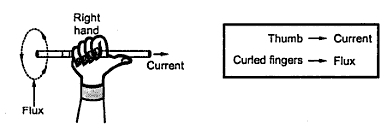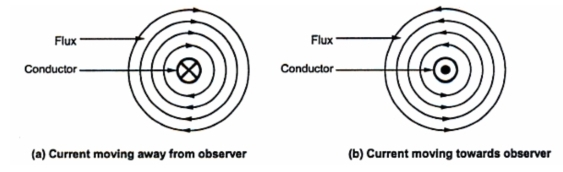### Revision of Electromagnetism

1. Revision of Electromagnetism
When a conductor carries a current, it creates a magnetic field around it. The direction of such magnetic field depends on the direction of the current passing through the conductor. So electric current and magnetism are very closely related to each other. This relationship plays an important role in the d.c. machines.
Let us see in brief, the role to determine the direction of the flux produced by a current carrying conductor.
1.1 Right Hand Thumb Rule
It states that ''Hold the current carrying conductor in the right hand such that the thumb is pointing in the direction of current and parallel to the conductor, then curled fingers point in the direction of the magnetic field or flux around it''.
The Fig 1. explains the rule.Fig.  1
Conventionally, such conductors are observed, assuming them to be placed perpendicular to the plane of the paper. So current moving away from the observer is denoted by a 'cross' while current coming towards the observer is denoted by a 'dot'. If now right hand is adjusted in such a way, that thew thumb is pointing in the direction of current denoted as 'cross' i.e. going into the paper, then curled fingers indicate the direction of flux as clockwise, as shown in the Fig.2(a). While if thumb of right hand is adjusted in the direction of current shown as 'dot' i.e. coming out of paper, then curled fingers indicate the direction of flux as anticlockwise as shown in the Fig. 2(b).Fig.  2
1.2 Magnetic Field due to Circular Conductor
Consider an arrangement in which along conductor is wound with number of turns in a core, close together to form a coil. This is called a solenoid as shown in the Fig. 3. When such a conductor carries a current, the magnetic field gets produced around the core.Fig. 3
Identifying the direction of flux and hence identifying the two ends of the core as N pole or S pole is important in understanding the principle of d.c. machine. The right hand thumb rule can be modified for such case as stated below,
The right hand thumb rule : Hold the solenoid in the right hand such that curled fingers point in the direction of the current through the curled conductor, then the outstretched thumb along the axis of the solenoid points to the North pole of the solenoid or points in the direction of flux lines inside the core.
This is represented in the Fig.4.Fig. 4

Key Point : The direction of flux can be reversed either by changing direction of current through the conductor by reversing the polarities of the supply or by changing the direction of winding of the conductors around the core.
With this background, let us start the detail study of a d.c. generator.